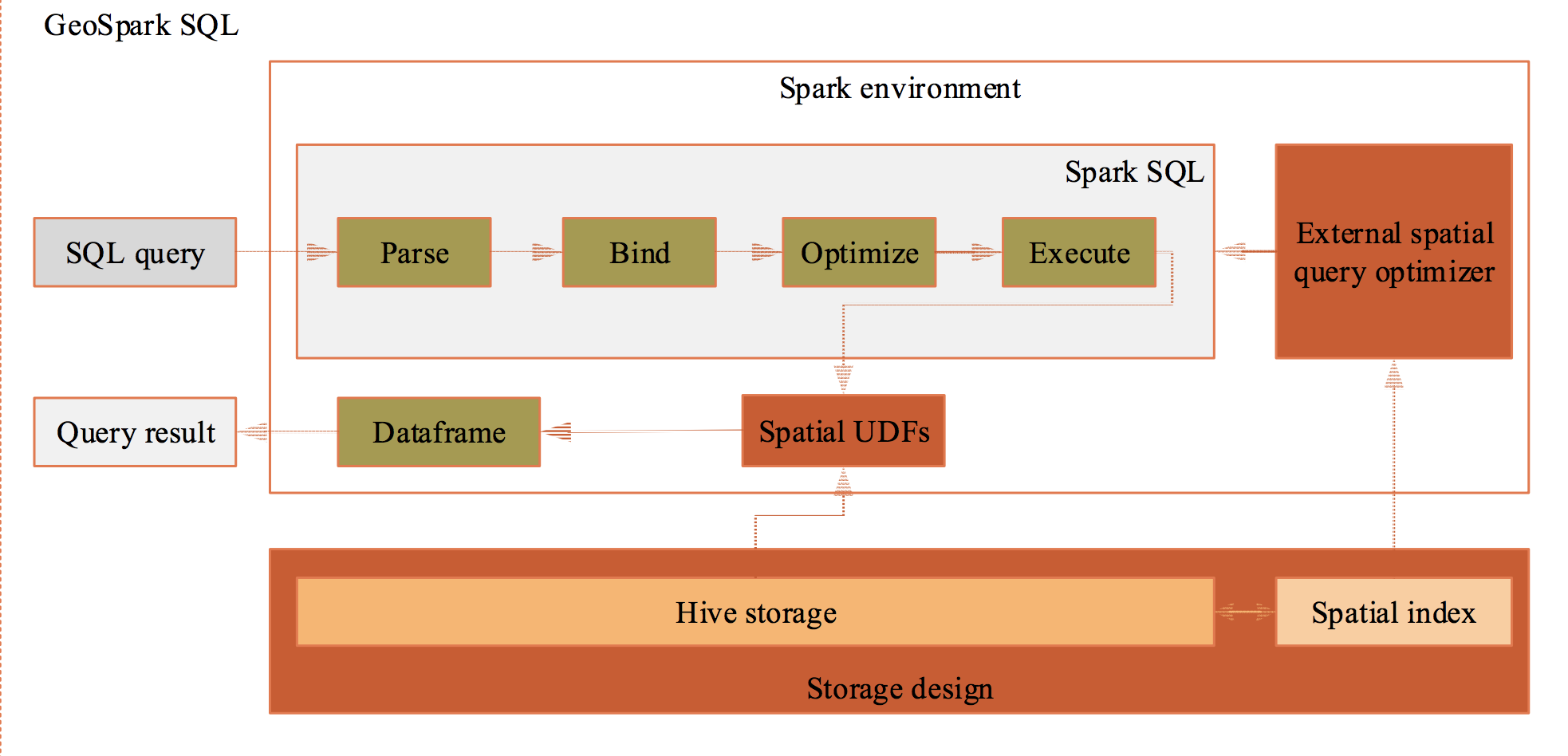Skip to content

# r-spark/geospark forked from harryprince/geospark

bring sf to spark in production
R
This branch is 1 commit ahead, 8 commits behind harryprince:master.Fetching latest commit…
Cannot retrieve the latest commit at this time.
Type Name Latest commit message Commit time
Failed to load latest commit information.Rdocsinst/examplesmantests.Rbuildignore.Rhistory.gitignore.travis.ymlDESCRIPTIONNAMESPACEREADME.mdReference.mdgeospark.Rproj

# GeoSpark: Bring sf to spark## Introduction & Philosophy

Goal: make traditional GISer handle geospatial big data easier.

The origin idea comes from Uber, which proposed a ESRI Hive UDF + Presto solution to solve large-scale geospatial data processing problem with spatial index in production.

However, The Uber solution is not open source yet and Presto is not popular than Spark.

In that, `geospark` R package aims at bringing local sf functions to distributed spark mode with GeoSpark scala package.

Currently, `geospark` support the most of important `sf` functions in spark, here is a summary comparison. And the `geospark` R package is keeping close with geospatial and big data community, which powered by sparklyr, sf, dplyr and dbplyr.

## Installation

This package requires Apache Spark 2.X which you can install using `sparklyr::install_spark("2.3")`, and spark2.4 is not supported yet; in addition, you can install `geospark` as follows:

`pak::pkg_install("harryprince/geospark")`

## Getting Started

In this example we will join spatial data using quadrad tree indexing. First, we will initialize the `geospark` extension and connect to Spark using `sparklyr`:

```library(sparklyr)
library(geospark)

sc <- spark_connect(master = "local")```

Next we will load some spatial dataset containing as polygons and points.

```polygons <- read.table(system.file(package="geospark","examples/polygons.txt"), sep="|", col.names=c("area","geom"))
points <- read.table(system.file(package="geospark","examples/points.txt"), sep="|", col.names=c("city","state","geom"))

polygons_wkt <- copy_to(sc, polygons)
points_wkt <- copy_to(sc, points)```

And we can quickly visulize the dataset by `mapview` and `sf`.

``````M1 = polygons %>%
sf::st_as_sf(wkt="geom") %>% mapview::mapview()

M2 = points %>%
sf::st_as_sf(wkt="geom") %>% mapview::mapview()

M1+M2
``````### The SQL Mode

Now we can perform a GeoSpatial join using the `st_contains` which converts `wkt` into geometry object. To get the original data from `wkt` format, we will use the `st_geomfromwkt` functions. We can execute this spatial query using `DBI`:

```DBI::dbGetQuery(sc, "
SELECT area, state, count(*) cnt FROM
(SELECT area, ST_GeomFromWKT(polygons.geom) as y FROM polygons) polygons
INNER JOIN
(SELECT ST_GeomFromWKT (points.geom) as x, state, city FROM points) points
WHERE ST_Contains(polygons.y,points.x) GROUP BY area, state")```
``````             area state cnt
1      texas area    TX  10
2     dakota area    SD   1
3     dakota area    ND  10
4 california area    CA  10
5   new york area    NY   9
``````

### The Tidyverse Mode

You can also perform this query using `dplyr` as follows:

```library(dplyr)
polygons_wkt <- mutate(polygons_wkt, y = st_geomfromwkt(geom))
points_wkt <- mutate(points_wkt, x = st_geomfromwkt(geom))

sc_res <- inner_join(polygons_wkt,
points_wkt,
sql_on = sql("st_contains(y,x)")) %>%
group_by(area, state) %>%
summarise(cnt = n())

sc_res %>%
head()```
``````# Source: spark<?> [?? x 3]
# Groups: area
area            state   cnt
<chr>           <chr> <dbl>
1 texas area      TX       10
2 dakota area     SD        1
3 dakota area     ND       10
4 california area CA       10
5 new york area   NY        9
``````

The final result can be present by `leaflet`.

``````Idx_df = collect(sc_res) %>%
right_join(polygons,by = (c("area"="area"))) %>%
sf::st_as_sf(wkt="geom")

Idx_df %>%
leaflet::leaflet() %>%
leaflet::addTiles() %>%
leaflet::addPolygons(popup = ~as.character(cnt),color=~colormap::colormap_pal()(cnt))

``````Finally, we can disconnect:

`spark_disconnect_all()`

## Performance

### Configuration

To improve performance, it is recommended to use the `KryoSerializer` and the `GeoSparkKryoRegistrator` before connecting as follows:

```conf <- spark_config()
conf\$spark.serializer <- "org.apache.spark.serializer.KryoSerializer"
conf\$spark.kryo.registrator <- "org.datasyslab.geospark.serde.GeoSparkKryoRegistrator"```

### Benchmarks

This performance comparison is an extract from the original GeoSpark: A Cluster Computing Framework for Processing Spatial Data paper:

No. test case the number of records
1 SELECT IDCODE FROM zhenlongxiang WHERE ST_Disjoint(geom,ST_GeomFromText(‘POLYGON((517000 1520000,619000 1520000,619000 2530000,517000 2530000,517000 1520000))’)); 85,236 rows
2 SELECT fid FROM cyclonepoint WHERE ST_Disjoint(geom,ST_GeomFromText(‘POLYGON((90 3,170 3,170 55,90 55,90 3))’,4326)) 60,591 rows

Query performance(ms),

No. PostGIS/PostgreSQL GeoSpark SQL ESRI Spatial Framework for Hadoop
1 9631 480 40,784
2 110872 394 64,217

According to this paper, the Geospark SQL definitely outperforms PG and ESRI UDF under a very large data set.

If you are wondering how the spatial index accelerate the query process, here is a good Uber example: Unwinding Uber’s Most Efficient Service and the Chinese translation version

## Functions

### Constructor

name desc
`ST_GeomFromWKT` Construct a Geometry from Wkt.
`ST_GeomFromWKB` Construct a Geometry from Wkb.
`ST_GeomFromGeoJSON` Construct a Geometry from GeoJSON.
`ST_Point` Construct a Point from X and Y.
`ST_PointFromText` Construct a Point from Text, delimited by Delimiter.
`ST_PolygonFromText` Construct a Polygon from Text, delimited by Delimiter.
`ST_LineStringFromText` Construct a LineString from Text, delimited by Delimiter.
`ST_PolygonFromEnvelope` Construct a Polygon from MinX, MinY, MaxX, MaxY.

### Geometry Measurement

name desc
`ST_Length` Return the perimeter of A
`ST_Area` Return the area of A
`ST_Distance` Return the Euclidean distance between A and B

### Spatial Joinname desc
`ST_Contains`
`ST_Intersects`
`ST_Within`
`ST_Equals`
`ST_Crosses`
`ST_Touches`
`ST_Overlaps`

### Distance join

`ST_Distance`:

Spark GIS SQL mode example:

``````SELECT *
FROM pointdf1, pointdf2
WHERE ST_Distance(pointdf1.pointshape1,pointdf2.pointshape2) <= 2
``````

Tidyverse style example:

``````st_join(x = pointdf1,
y = pointdf2,
join = sql("ST_Distance(pointshape1, pointshape2) <= 2"))
``````

### Aggregation

name desc
`ST_Envelope_Aggr` Return the entire envelope boundary of all geometries in A
`ST_Union_Aggr` Return the polygon union of all polygons in A

### More Advacned Functions

name desc
`ST_ConvexHull` Return the Convex Hull of polgyon A
`ST_Envelope` Return the envelop boundary of A
`ST_Centroid` Return the centroid point of A
`ST_Transform` Transform the Spatial Reference System / Coordinate Reference System of A, from SourceCRS to TargetCRS
`ST_IsValid` Test if a geometry is well formed
`ST_PrecisionReduce` Reduce the decimals places in the coordinates of the geometry to the given number of decimal places. The last decimal place will be rounded.
`ST_IsSimple` Test if geometry's only self-intersections are at boundary points.
`ST_Buffer` Returns a geometry/geography that represents all points whose distance from this Geometry/geography is less than or equal to distance.
`ST_AsText` Return the Well-Known Text string representation of a geometry

#You can’t perform that action at this time.